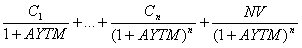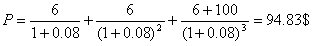Chart Pro online
User name (email):

 Recently used

 Forex

 Indices

# Bonds

Bonds are debt. Bond obliges the issuer to pay the amount of the nominal value of bond and the contractual interest, to the person designated in the bond.

Bond contains various data: tag of bond, issuer and buyer data, the nominal value, the annual interest rate, maturity date, control serial number, etc. Bonds can also contain coupon rate. Coupon contains data for the payment of interest: the amount of interest, maturity date, place and manner of payment, serial number, coupon number, etc.

Bonds are fixed-income securities, because you know exact amount of money which you will collect if you hold it till maturity date.

Bonds generally have four different kinds of yield: coupon interest rate, one period rate of return, current yield and the anticipated yield to maturity.

Coupon interest rate is printed on the coupon and never change.

One period rate of return is rate of return for the period of holding bonds:

Rate of return = (gain or loss in the period of holding bonds + coupon interest rate) / purchase price at the beginning of the period of possession

Current yield shows the ratio of annual payments to the current market price of bonds.

Current yield = Annual coupon payments / Current market price

Anticipated yield to maturity (AYTM) can be calculated using the formula for current value:

 Current value =## Determining the value of bonds

Each bond has a nominal value, that value will be paid to the owner on the date of maturity. Market value is the current value of the bond formed through the process of supply and demand.

Current value of zero coupon bond can be calculated using the formula:P - the current market value of bond
NV - nominal value of bond
r - yield to maturity
n - a period of time to maturity

Current value of bond with coupons could be calculated discounting of future periodic income from interest and principal on date of maturity:C - annual coupon payments

Example: let's say that current interest rate is 8% (r), nominal value 100\$, interest rate with a maturity period of three years is 6%. The current value of the bonds is: